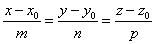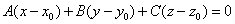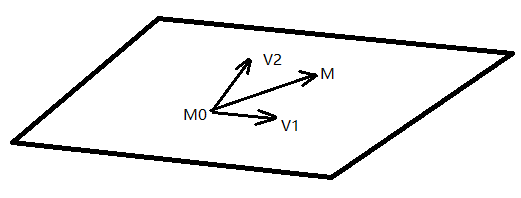# eryar

posts - 435, comments - 588, trackbacks - 0, articles - 0gp_Lin (const gp_Pnt &P, const gp_Dir &V) 即通过点和方向来构造直线。由直线的点向式方程容易导出直线的参数方程：```void IntAna_IntConicQuad::Perform (const gp_Lin& L, const gp_Pln& P,
const Standard_Real Tolang,
const Standard_Real Tol,
const Standard_Real Len) {
// Tolang represente la tolerance angulaire a partir de laquelle on considere
// que l angle entre 2 vecteurs est nul. On raisonnera sur le cosinus de cet
// angle, (on a Cos(t) equivalent a t au voisinage de Pi/2).

done=Standard_False;
Standard_Real A,B,C,D;
Standard_Real Al,Bl,Cl;
Standard_Real Dis,Direc;
P.Coefficients(A,B,C,D);
gp_Pnt Orig(L.Location());
L.Direction().Coord(Al,Bl,Cl);
Direc=A*Al+B*Bl+C*Cl;
Dis = A*Orig.X() + B*Orig.Y() + C*Orig.Z() + D;
//
parallel=Standard_False;
if (Abs(Direc) < Tolang) {
parallel=Standard_True;
if (Len!=0 && Direc!=0) {
//check the distance from bounding point of the line to the plane
gp_Pnt aP1, aP2;
//
aP1.SetCoord(Orig.X()-Dis*A, Orig.Y()-Dis*B, Orig.Z()-Dis*C);
aP2.SetCoord(aP1.X()+Len*Al, aP1.Y()+Len*Bl, aP1.Z()+Len*Cl);
if (P.Distance(aP2) > Tol) {
parallel=Standard_False;
}
}
}
if (parallel) {
if (Abs(Dis) < Tolang) {
}
else {
}
}
else {
parallel=Standard_False;
nbpts = 1;
paramonc  = - Dis/Direc;
pnts.SetCoord(Orig.X()+paramonc*Al,
Orig.Y()+paramonc*Bl,
Orig.Z()+paramonc*Cl);
}
done=Standard_True;
}```